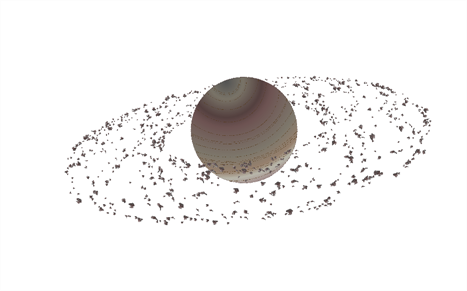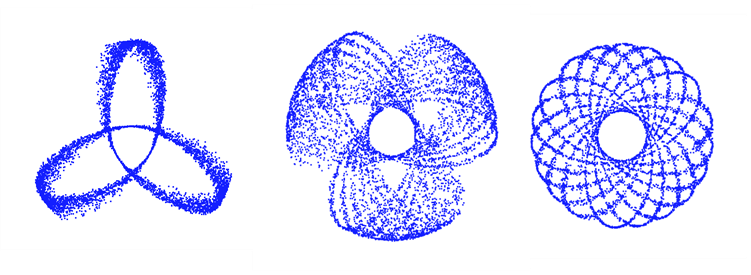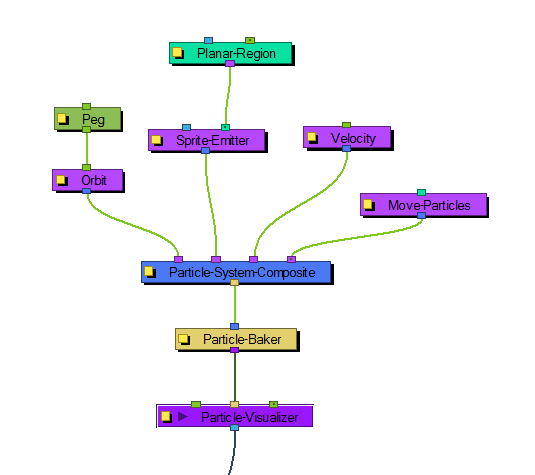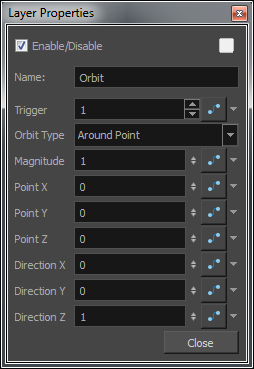# OrbitThe Orbit effect lets you define an axis the particles will be attracted to and orbit around. You can place a Peg layer into the orbit to move the position of the orbit without adjusting the parameters in the Layer Properties.

Fine-tuning the Orbit effect can take a bit of finesse because the orbit takes into account the velocity of the particles, and uses this to determine whether:

 • The particle will orbit around the axis
 • The particle will be influenced but eventually escape
 • The particle will hardly be influenced at all.

This is just like how gravity between astral bodies works in space. Here are some examples of how having the orbit in the wrong place can give some cool (but perhaps not useful) effects:You will also want to make sure that your initial velocity is varied, so use an interpolated speed and set two different values. That way, there will be a spread between them. If not, then you will get the effects as shown above.

Also, you should use the Peg layer to move the position of the Orbit node around until you find the right spot to get the look you're trying to achieve. It also helps to set some pre-roll frames on your Baker to get things ramped-up before you start to manipulate the position of the Orbit node.

Here's an example of what a typical node structure with an Orbit node looks like:### Orbit Properties

 Parameter Description Name Allows you to change the name given to the node. Trigger An on/off switch using binary code. 1 = on, 0 = off. Orbit Type Lets you select the type of Orbit you want to use. For most scenarios, like asteroids orbiting a planet, a point will suffice. If you want to rotate around an axis instead, choose between the X, Y, Z axes or set up your own custom axis. Magnitude Adjust the amount that the acceleration of each particle will be adjusted. The higher the value, the more the particle will accelerate towards the orbit. A magnitude of 0.001 is a good number to use for this. When the magnitude is set too high, the particles will simply fly out into space. Point X Defines the X position of the centre of the Orbit. You may also leave this at 0 and use a peg to move the position of the Orbit node. Point Y and Z Defines the Y and Z position of the centre of the Orbit. Direction X Defines the X component of the axis of rotation for the orbit. This is only taken into account if you choose to set up a custom axis. If this is set to 1, and Y and Z are set to 0, then the axis will be going in the same direction as the X axis. If the X component is set to 1, and the Y component is also set to 1, then the axis will be at a 45 degree angle between the two. Direction Y and Z Defines the Y and Z components of the axis of rotation for the orbit.